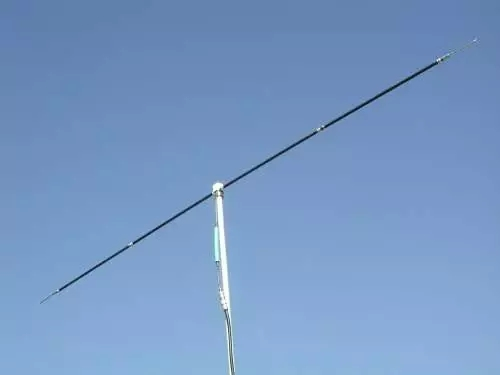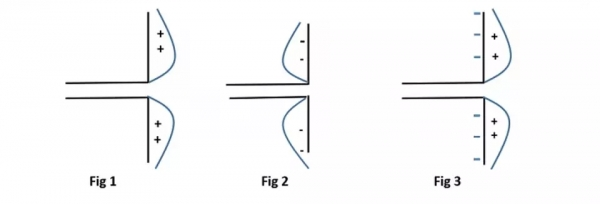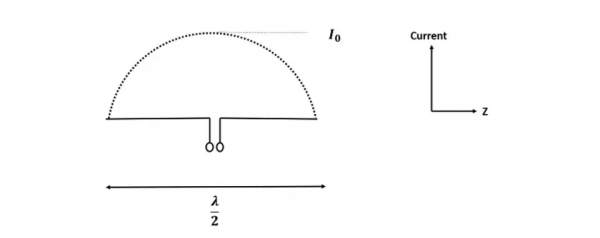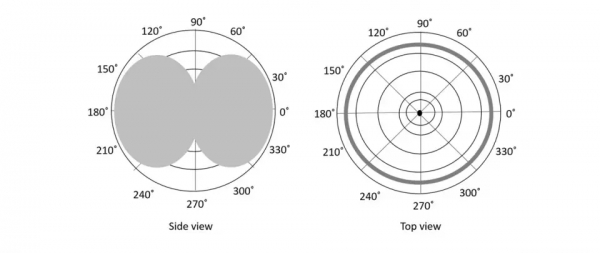# What is a Half Wave Dipole Antenna?

The dipole antenna is a widely popular antenna that is used mostly for the reception. The dipole antenna is cut and bent for effective radiation. The length of the total wire, which is being used as a dipole, equals half of the wavelength (i.e., l = λ/2). Such an antenna is called as a half-wave dipole antenna, also known as Hertz antenna. This antenna which is mostly used in radio receivers operates is around 3KHz to 300GHz.## Construction & Working of Half-Wave Dipole

The edge of the dipole has maximum voltage. This voltage is alternating (AC) in nature. At the positive peak of the voltage, the electrons tend to move in one direction and at the negative peak, the electrons move in the other direction.The figures given above show the working of a half-wave dipole.

• Fig 1 shows the dipole when the charges induced are in the positive half cycle. Now, the electrons tend to move towards the charge.

• Fig 2 shows the dipole with negative charges induced. The electrons here tend to move away from the dipole.

• Fig 3 shows the dipole with next positive half cycle. Hence, the electrons again move towards the charge.

The cumulative effect of this produces a varying field effect which gets radiated in the same pattern produced on it. Hence, the output would be effective radiation following the cycles of the output voltage pattern. Thus, a half-wave dipole radiates effectively.The above figure shows the current distribution of half wave dipole. The directivity of half wave dipole is 2.15dBi, which is reasonably good. Where ‘i’ represents the isotropic radiation.

The radiation pattern of this half-wave dipole is Omni-directional in the H-plane. It is desirable for many applications such as mobile communications, radio receivers etc.

The figure below indicates the radiation pattern of a half wave dipole in both H-plane and V-plane.The radius of the dipole does not affect its input impedance in this half wave dipole because the length of this dipole is a half wave and it is the first resonant length. An antenna works effectively at its resonant frequency, which occurs at its resonant length.

• Input impedance is not sensitive
• Matches well with transmission line impedance
• Has reasonable length
• Length of the antenna matches with size and directivity